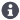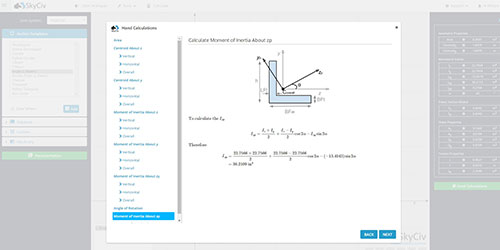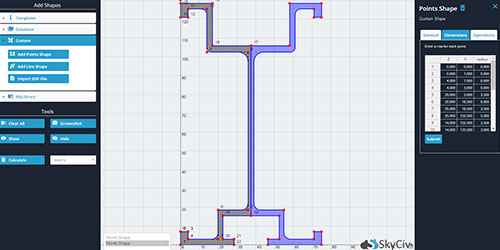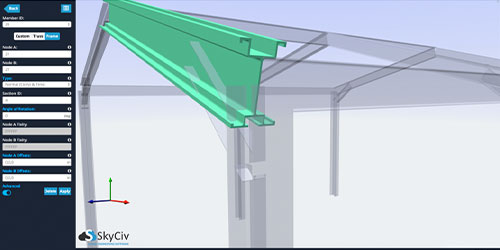# Free Moment of Inertia Calculator / Centroid Calculator

## How to use this Moment of Inertia Calculator

This simple, easy-to-use moment of inertia calculator will find the moment of inertia of a circle, rectangle, hollow rectangular section (HSS), hollow circular section, triangle, I-Beam, T-Beam, L-Sections (angles) and channel sections, as well as centroid, section modulus and many more results.

You can solve up to three sections before you're required to sign up for a free account - which also gives you access to more software and results.

To start using the free centroid calculator to calculate moment of inertia, simply choose the cross section you want to evaluate from the drop down list, then enter in the dimensions and click Calculate. The results for centroid, moment of inertia, statical moment section modulus and torsion constant will display on your right.

## SkyCiv Moment of Inertia Calculatorb:
h:

Units:

Notation Value Unit
A- -
Iz- -
Iy- -
Izp- -
Iyp- -
αp- -
Cz- -
Cy- -
Qz- -
Qy- -
Sz- -
Sy- -
J- -## Need More Functionality?

###### Upgrade to a professional plan to unlock full features## About the Moment of Inertia Calculator

#### Find the Moment of Inertia with ease

This free multi-purpose calculator is taken from our full suite Structural Analysis Software. It allows you to:

• Calculate the Moment of Inertia (I) of a beam section (Second Moment of Area)
• Centroid Calculator used to calculate the Centroid (C) in the X and Y axis of a beam section
• Calculate the First moment of area (Statical Moment of Inertia) (Q) of a beam section (First Moment of Area)
• A Section Modulus Calculator to calculate the Section Modulus (Z) of a beam section
• Calculate the Torsion Constant (J) of a beam section

More information on these values is provided under "Section Properties Explained"

#### Moment of Inertia

For instance, if you're looking how to calculate the moment of inertia of a rectangle you can use the tool above simply by selecting rectangle from the drop down list then entering some dimensions for height and width (e.g. 100, 200). After clicking "Calculate", the tool will calculate the moment of inertia. Our paid version will show the full hand calculations of how the tool got to this result. These detailed step-by-step instructions help for you to follow the calculations and learn how to calculate the moment of inertia. Alternatively, we have a tutorial on how to find moment of inertia.

#### Section Modulus Calculator

The calculator will also produce results like section modulus (Z) and the statical moment of inertia (Q) for your sections. Finally, the result for Torsion Constant (J) will also display using this tool. The SkyCiv Section Builder will also produce these results accurately using FEA analysis of the cross section. So if you need more results, or to add custom shapes - please check out our extra functionality of the SkyCiv Section Builder.

#### Section Properties Explained

• The moment of inertia calculator will accurately calculate a number of important section properties used in structural engineering, including:
• Area of Section (A) - Section area is a fairly simple calculation, but directly used in axial stress calculations (the more cross section area, the more axial strength)
• Moment of Inertia (Iz, Iy) – also known as second moment of area, is a calculation used to determine the strength of a member and it’s resistance against deflection. The higher this number, the stronger the section. There are two axis here:
• Z-Axis (Iz) – This is about the Z axis and is typically considered the major axis since it is usually the strongest direction of the member
• Y-Axis (Iy) – This is about the Y axis and is considered the minor or weak axis. This is because sections aren’t designed to take as much force about this axis
• Also worth noting that if a shape has the same dimensions in both directions (square, circular etc..) these values will be the same in both directions. See Moment of Ineria of a circle to learn more.
• Centroid (Cz, Cy) – this is the center of mass for the section and usually has a Z and Y component. For symmetrical shapes, this will be geometric center. For non-symmetrical shapes (such as angle, Channel) these will be in different locations. Learn how to calculate the centroid of a beam section
• Statical Moment of Inertia (Qz, Qy) – Also known as First Moment of Area, this measures the distribution of a beam section’s area from an axis. Like the Moment of Inertia, these are in both the Z and Y direction. These are typically used in shear stress calculations, so the larger this value the stronger the section is against shearing. The calculator will provide this value, but click here to learn more about calculating the first moment of area.
• Elastic Section Modulus (Sz, Sy) – Also known as statical section modulus, and are used in bending stress calculations. They are usually calculated to the top and bottom corner fibres from a particular axis. For instance, Szt is the section modulus about Z to the top fibre. For the moment calculator above, this is taken to the top fibres by default.
• Torsion Constant (J)
• Other Parameters – These are calculated by the full SkyCiv Section Builder:
• Product of Inertia (about Z and Y Axis)
• Angle of Rotation of Principle Axis
• Radius of Gyration (about Z and Y Axis)
• Plastic Section Modulus (about Z and Y Axis)
• Plastic Neutral Axis (about Z and Y Axis)
• Shear Area (about Z and Y Axis)
• Distance of Shear Centre to Centroid (in both Z and Y Axis)
• Torsion Constant (Using FEA)
• Warping Constant
• Monosymmetry Constant (about Z and Y Axis)